Home

A First Look at Mechanics

Chapter 1

Time

Mark Twain once remarked that everybody complains about the weather but nobody does anything about it! His idea was of course that no one can do anything about the weather. This appears to be even more so in the case of time. If we represent space as a straight line, then we can go backward or forward on the line. In 2 dimensions we can move around in a plane. In 3 dimensions we can move in any direction whatsoever. But time is different. Like pieces of cork on the surface of a stream, we are swept along into the future at a constant pace. Science fiction has long toyed with the idea of going backward in time. But In reality nobody can go back in time (fortunately, if you think of some of the logical consequences if one could). In theory it is possible to go forward in time. But at present that is feasible only for subatomic particles. No one seriously anticipates human beings leapfrogging ahead in time … at least not anytime in the foreseeable future. For our purposes here, time flows along at a constant, immutable rate, and we (and everything in the physical universe) are caught up in its steady pulse. Although we might be interested in (and might even venture to predict) what the future holds for us and the world, the simple reality is that we just have to wait until the future arrives to find out for sure.

Time is one of the most fundamental quantities in physics. And the amazing thing is that all of the world’s scientists have agreed that they will measure this one particular quantity using the same, common unit (namely the second). Space, another fundamental quantity of great importance, is measured in feet, meters, light years, and lots of other ways. But scientists everywhere agree that the way to count time is in seconds, or at least in multiples and/or fractions of the second.

But what other units are based on seconds? Well, a minute consists of 60 seconds. And an hour consists of 60 minutes. Therefore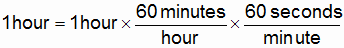(1_1)

Note the trick used in Eq. 1_1 in order to convert an hour into seconds. On the right side of the equation we simply multiplied 1 hour by 1 (60 minutes divided by 1 hour equals 1) and then by 1 again. On the right side of the equation, hour cancels hour, minutes cancel minute, and the answer is in seconds! You can use this trick again and again to convert from one physical unit into another.

Now physics is called one of the exact sciences, which means that its predictions can be tested by making careful measurements. How do we measure time? Why, with a clock of course! If our clock has a second hand, then it’s a simple matter to count the seconds as they tick by. For example, if we are measuring the time it takes for a coin to drop from a state of rest to the ground 10 meters below, then we might say that t1 is whatever the second hand of our clock (or watch) points to when the coin is released, and t2 is whatever the second hand points to when the coin hits the ground. The elapsed time … the length of time the coin falls … is then t2 - t1.

But what happens if the coin hits the ground sometime between 2 consecutive ticks? Obviously the total elapsed time might then contain not only a whole number of seconds, but also some fraction of a second. In order to deal with such contingencies, physicists have divided the second into fractions. For example, 1 millisecond consists of 1 thousandth of a second! 1 microsecond consists of a millionth of a second! (Yes, in modern times there are ways of accurately measuring such very short times.)

There is one problem with measuring time which warrants mention, and that is that we believe we are accurately measuring time with a clock because it seems to us that the ticks occur at regular and repeating intervals. Since the clock is a physical device, this is tantamount to a belief that certain processes in nature repeat at a constant rate. How do we know that is the case? Because it seems that way. No one has ever figured out a more concrete basis for our belief that time can be measured.

In conclusion, the second is the fundamental unit of time. We can lump seconds into longer times … minutes, hours, days … or we can divide a second into smaller units … milliseconds, microseconds and so on. And we can specify the time of a particular event using subscripts. Typically we might simply use numeric subscripts … t1, t2, etc., as above. But in truth we can use any subscripts that are meaningful. In the problem above we might have denoted the two instants in time as treleased and tground.

Chapter 2

Space

"Space … the final frontier!" Words made famous by the science fiction TV program, "Star Trek." Space is different from time in at least one important respect. Whereas we are swept along at a steady pace through time, we have a good deal of control over how we move through space. We can go in various directions, and we can move through space at various speeds. We can define "forward" and "backward" and other directions to our liking. These directions may be arbitrary and convenient for the user. For example, viewed from "outer space," "up" for a person at one point on the Earth would be "down" for someone halfway around the globe.

Here again one of the physicist’s primary concerns is measurement. How shall we specify points in space? In this text we shall concentrate upon phenomena in 2-dimensions. A good approximation to a 2-dimensional space would be a tabletop or even a limited area of the Earth’s surface. The question then becomes, how do we specify points in a 2-dimensional space, which is to say in a plane? We shall consider part of a desktop or tabletop.

Let’s lay a sheet of graph paper on the tabletop. An array of small squares is printed on the paper. Select a horizontal line through the middle of the sheet, and make it stand out by drawing your own line in pencil or pen over the existing line. Now select a vertical line down the middle of the paper. Emphasize this line too by drawing your own line on top of it. At the right end of the horizontal line, print "x" for "the x axis." And at the top of the vertical line print "y" for "the y axis." You end up with something like the drawing in Fig. 2_1.

Figure 2_1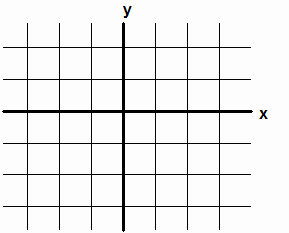An xy Coordinate System

Now although it is the lines in Fig. 2_1 that stand out, with a little imagination you can see that there are many well-defined points in the drawing. Everywhere two lines … a horizontal and a vertical one … intersect, there is a unique point. Indeed there are 42 unique points in the drawing. The point where the x and y axes intersect is called the Origin. Note that all of the other points can be uniquely specified by counting how many squares we must move to the right or left, and how many points we must then move up or down, in order to get from the Origin to a given point. Indeed, in Fig. 2_2 we can attach a number to each point on the x axis, and another number to each point on the y axis. Note how the points to the right of the Origin are identified by positive numbers. Similarly for points above the Origin. Points to the left of the Origin, and below the Origin, are identified using negative numbers.

Figure 2_2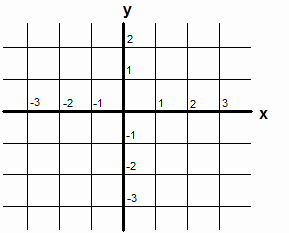xy Coordinate System with Subdivided Axes

Figures like Fig. 2_2 are called "rectangular" coordinate systems because they are divided into a grid of rectangles (squares in our particular case). Note how any intersection point in the grid can now be identified by a pair of numbers. These two numbers are called the point’s x and y Displacement components (the "Displacement" being from the Origin). For example, in Fig. 2_3 the Displacement components of point D1 are (2,1). That is, in order to get to D1 from the Origin, we undergo a displacement of 2 subdivisions to the right, followed by a displacement of 1 subdivision upward. Similarly, the Displacement components of point D2 are (-3,-2).

Quantities that are expressed as 2 or more numbers are called vectors, and the numbers are called the vector’s components. We shall identify vectors in text using bold type, and vector components by enclosing them in parentheses. Note that vectors and/or their components contain more information than quantities like instants in time, which are expressed as single numbers.

Figure 2_3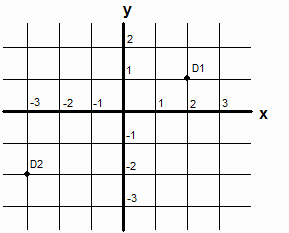2 Points in a Rectangular Coordinate System

Now here is a limitation of the coordinate system points in Fig. 2_3. There are actually an infinite number of possible points in the xy plane. Out of that number we have provided a means of uniquely identifying only 42 points, namely those at which horizontal and vertical lines intersect. Fig. 2_4 shows a point D3. Note how this point does not lie on the intersection of a vertical and a horizontal line.

Figure 2_4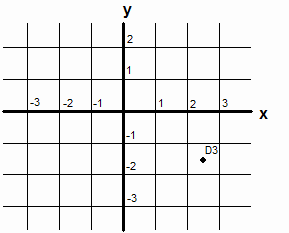Point D3 … How Shall we Uniquely Specify It???

As you can see, point D3 in Fig. 2_4 appears to lie in the center of one of the grid’s squares. And as you might guess, its Displacement components can be uniquely specified by the x and y component pair, (+2 ½, -1 ½), or by (2.5, -1.5).

Well, we are almost done with our discussion of coordinate systems. One small piece of unfinished business remains. Assuming the coordinate system corresponds to a plane in real space, how much space does one subdivision to the right or left, and/or one subdivision up or down, correspond to? Does the side of a square represent an inch? Or does it perhaps represent a mile? Let’s say a square’s side represents a meter. In order to make that clear to all who look at a drawing of the coordinate system, it’s customary to place the units of each axis in parentheses following the axis label. Fig. 2_5 illustrates. Now we are done! We can represent how many meters any given point is displaced to the right or left of the y axis, and how many meters the same point is displaced above or below the x axis. If the point doesn’t lie right on the intersection of 2 lines, then we can use fractions. We can uniquely define any point in 2 dimensional space with just 2 components!

Figure 2_5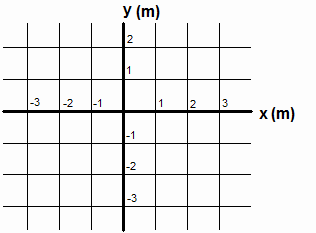x and y axes Labeled with Units of Measure

One final point about space warrants mention, and that is that its measurement doesn’t seem to be quite as intuitively based as the measurement of time. For example, if we lay two sticks side by side and if their two endpoints coincide, then few of us would doubt that they measure a line twice as long as either one alone does when they are laid end to end. They certainly seem to do so; but our intuition is backed to some extent by logical reasoning. The ancient Greeks were aware of this and built up an entire "science" about space without considering time at all. The name of that science is "Geometry," which literally means "measurement of the Earth."

Chapter 3

Changes of D (Intervals in Space)

At this point we have learned how to uniquely specify Displacements from the Origin (i.e., positions or points in space). A problem of great interest in physics is how objects move through space. Physicists have long used the Greek letter "D" (pronounced "delta") to signify "change in." Thus a change in an object’s Displacement would be called "delta D" (or simply DD for short). Fig. 3_1 shows 2 points, Di and Df. (The subscript "i" stands for "initial", and the subscript "f" stands for "final".) The object is initially at Di, and it is finally at Df. Notice the arrow. It suggests that the object has gone from point Di to Df.

Figure 3_1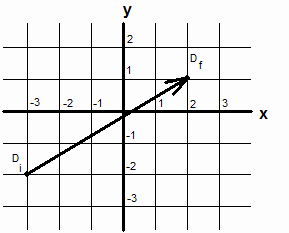A Change in Displacement

Now we could simply state the components of Di and Df in order to describe the change in displacement. That would contain all of the information. However, that would also be focusing upon the start and end points, and not on the change itself. Rather than do that, let’s invent a new pair of numbers called the components of DD. We define DD’s x component to be simply the x component of point Df minus the x component of point Di. Similarly, DD’s y component is defined to be the y component of Df minus the y component of Di. If we symbolize the actual change as DD, then we have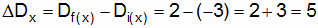, (3_1)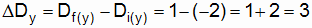. (3_2)

Like D, DD is a vector … it has 2 components.

There is a good deal of useful information in DD’s components. Note first of all that the x component of DD is positive (+5). That indicates that, in going from Di to Df (or from the vector’s tail to its head) we have moved in the positive x direction. Similarly, the positive sign of DD’s y component indicates that we have moved in the positive y direction.

A second useful piece of information can be gleaned from the components of DD. As you have perhaps learned, the Pythagorean theorem in geometry states that the length of any right triangle’s hypotenuse equals the square root of the square of one side added to the square of the other side. Fig. 3_2 depicts how we can use this theorem to determine the length of the DD vector in Fig. 3_1.

Figure 3_2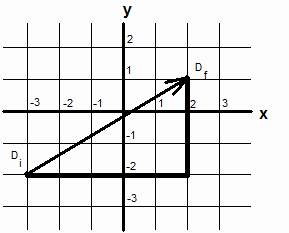Length of DD is the Hypotenuse of a Right Triangle

As you can seen in Fig. 3_2, the "x leg" (or the x component) of DD has a length of 5, which is just what we obtained in Eq. 3_1. Similarly, the "y leg" (or the y component) of DD has a length of 3 (see Eq. 3_2). If we signify the length of DD as simply "DD" (no bold type), then according to the Pythagorean theorem,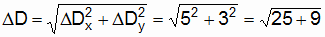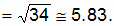(3_3)

Notice the symbol just in front of the final result of 5.83. It symbolizes "approximately equals." Your calculator might indicate a more complete result of 5.830951895 for the square root of 34 (but even that is not the full number).

One of the nice things about the length (or "magnitude") of a vector is that it is always positive. That is because a negative number (or negative component) squared is always positive. For example, Fig. 3_4 shows another DD vector. Its x and y components are both negative. But its magnitude is the square root of 32.

Figure 3_4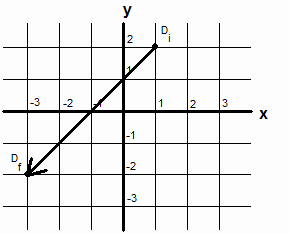A DD Vector with Negative Components

There are a few vectors in physics that have a special name for the magnitude or length of the vector. DD is one of those vectors. The magnitude of a DD vector is called the distance between the two end points.

Chapter 4

Motion

Much of physics is concerned with the motion of material objects. Physicists want to know, "How do objects move?" and "Why do they move as they do?" Motion of course occurs in space and time … it is the association of changes of position in space with intervals of time. In the simplest case motion is described as distance divided by time. It is often expressed as distance per unit of time, for example miles per hour or meters per second.

A useful way to graphically depict motion is to plot the distance along a vertical axis, as a function of the independent variable time (plotted on a horizontal axis). By "function" it is meant that for every instant of time on the horizontal axis, there is one and only one point in space (i.e. on the plot). Loosely speaking time is the independent variable because it flows by at a fixed rate. The distance traveled in a unit of time, on the other hand, will vary from case to case, and even from moment to moment in any given case.

Fig. 4_1 displays 2 oscillations along the x axis (just the kind of phenomenon we might use to measure time). At some instant t=0 a particle is at the origin (i.e., at x=0) on the x axis of a rectangular coordinate system. As time passes, the particle moves out to some maximum value on the positive x axis. It then stops and moves back in the negative x direction. Later still it comes to rest at a point on the negative x axis, and then moves back in the positive x direction. The motion is described as oscillatory because it occurs back and forth between two points and it repeats in a regular fashion. (Note that the plotting software has opted to put the axis labels, x and t, to the left of the vertical axis and beneath the horizontal axis.)

Figure 4_1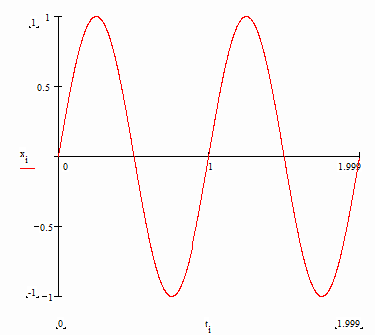Oscillation Along the x Axis

There is a good deal of information that can be gleaned from a graph such as that in Fig. 4_1. For example, it’s clear that the particle initially moves in the positive x direction. And it’s also clear that, in the first quarter of either cycle, the particle initially covers more distance during a small interval of time (say 1/16th of a cycle) than it does during a succeeding, equal-length interval. For example, at t=0 the particle is moving with its maximum speed in the positive x direction. A 16th of a cycle time later it has slowed down somewhat. Finally, where the first horizontal tangent to the curve occurs (i.e., at the end of the first quarter cycle), the particle has come to a halt.

Of course motion need not be oscillatory. Figure 4_2 plots the motion of an object dropped from a height of 1000 meters. Note how the number of meters per second, or the object’s speed, steadily increases as the object falls downward in the Earth’s gravitational field.

Figure 4_2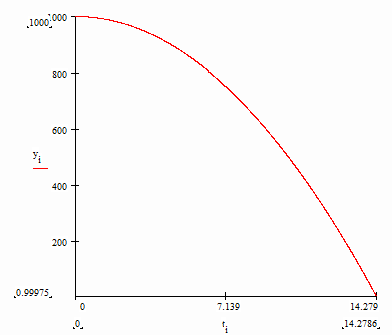Free Fall in the Earth’s Gravitational Field

In the next chapter we shall consider how to express motion quantitatively … a "science" known as kinematics. It is at the heart and soul of much of physics and was pioneered by such giants as Galileo (on whose shoulders the great Isaac Newton humbly claimed to have stood when he figured out the laws of mechanics).

Chapter 5

Average Velocity

Just as DD specifies a change in Displacement, so does velocity specify the rate at which such a change occurs. Average Velocity is defined to be a finite change in Displacement divided by the finite interval of time during which the change occurs. Since DD is a vector with 2 components, so is average velocity. Average velocity can usually be measured, since we are dealing with finite intervals of space and time.

By way of example, Fig. 5_1 shows a DD that points at an angle to both the x and y axis. The distance traveled is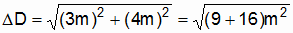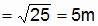. (5_1)

Figure 5_1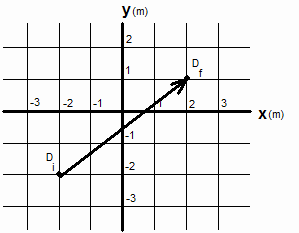A Change of Displacement in the xy Plane

Let’s call the interval of time that elapses, between the time an object is at Di until it is at Df, "Dt." The average velocity is then defined to be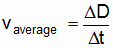. (5_1)

Now it is clear that the same DD can result in different average velocities. In the example, if Dt=5 seconds, then vaverage=1 meter/second. On the other hand, if Dt=10 seconds, then vaverage=.5 meter/second. And of course if Dt=.5 seconds, then vaverage=10 meter/second. For a fixed DD, the size of vaverage depends on how much time elapses.

We have become so accustomed to watching things constantly in time that a shortcoming of average velocity may not immediately be obvious. But let us for the moment imagine that we can only make discrete observations of where an object is, with no knowledge of its whereabouts between such observations. In Fig. 5_1 we shall imagine that our knowledge of the object’s position is limited to the two displacements (the tail and head of the arrow) shown. For all we know, between the times of our initial and final observations the object could have shot off to some enormous value of y and then returned again to point Df. Clearly its detailed motion, were we to know what it is, might be different than if the object had smoothly moved from point Di to point Df.

A solution to this problem lies in observing the object’s position more frequently (as we generally do with our eyes in real life). That amounts to making Dt smaller and smaller between successive observations. And this will of course lessen the distance traveled between successive observations. Ideally we would make Dt infinitesimally small, which for practical purposes means we would note the object’s position constantly in time.

Chapter 6

Velocity

"Instantaneous velocity" (usually referred to simply as "velocity") is the "average" velocity that would be measured when the time between successive position observations is infinitesimal, which is to say practically zero. In other words, it is as if we make an infinite number of position observations over the course of any finite interval of time (say 1 second). Experimentally that is generally not feasible. The power of the idea lies in the fact that calculus shows us how to calculate such values from the equations and laws of physics.

The idea of a Dt that is practically zero might take a bit of getting used to. One might at first conclude that a change in displacement, divided by a number that is practically zero, would result in a huge velocity. However, we must remember that as we decrease the size of Dt (the interval of time between successive position observations) we are also decreasing the distance the object travels. And one very small number divided by another very small number might result in a perfectly finite result.

If you take a course in calculus, you will study ratios of infinitesimal numbers. By way of example, let us use a previously considered motion, namely that of an object falling in the Earth’s gravitational field. For convenience, Fig. 6_1 repeats the plot of height (y coordinate) vs. time.

Figure 6_1Height vs. Time, Object in Free Fall

By way of review, let’s calculate the object’s average velocity during the time interval 0<t<14.279 seconds. (t=0 is the time the object is released from a state of rest, and t=14.279 seconds is the time it hits the ground.) Dy in this case is 1000 meters. And Dt is 14.279 seconds. Thus the average velocity has a magnitude (or speed) of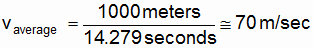. (6_1)

Let us now consider the infinitesimal time interval 0<t<dt, where "dt" is the calculus notation for "infinitesimal Dt." It is clear from experience (and from the plot) that the object can't have picked up much speed in any adequately short interval of time following its release. Indeed in an infinitesimal amount of time the object is still practically at rest. And a practically zero velocity is certainly different from the 70 meters/sec average velocity calculated in Eq. 6_1,

By the same token, the speed just before the object hits the ground (and comes to a sudden halt) is considerably more than the average 70 meters/sec. It is, in fact, 140 meters/sec. What we have here is an object which is initially at rest, but whose speed steadily increases so that v varies all along the free fall path. During any tiny time interval 0<t<t+dt<14.279 sec, v has a unique value. Since dt is infinitesimal, it is common practice to speak of v right at the instant t. However, it is worth always bearing in mind that any measurement of v actually entails a knowledge of 2 consecutive positions, however closely in time they might be assumed to be.

In 2 dimensions velocity could be in any direction in the plane. Its definition is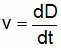. (6_2)

Here dD is the infinitesimal change in displacement that occurs in the infinitesimal time interval dt. Like other vectors, velocity has 2 components (for motion in a plane).

In Fig. 6_1 D was plotted, as a function of time, for a free-falling particle. It turns out that the magnitude of D can be expressed in terms of t in equation form: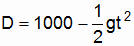. (6_3)

(Here D=1000 corresponds to the height at which the particle is released. g is a constant known as the acceleration of Earth’s gravity.) If you are familiar with calculus, you will see from Eq. 6_3 that dD/dt (i.e., v) is then just equal to gt. Physics leans so heavily upon calculus that a first course in physics and a first course in calculus are often taught concurrently.

Chapter 7

Acceleration

Just as velocity is the time rate of change of displacement (or position), so is acceleration the time rate of change of velocity. Let us imagine that we could make 3 measurements of a particle’s position, say D1, D2 and D3 at times t1, t2 = t1 + dt, and t3 = t2 + dt. We know that the velocity at time t1 + dt/2 is (D2 - D1)/dt and the velocity at time t2 + dt/2 is (D3 – D2)/dt. Then the acceleration at time t2 is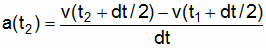. (7_1)

Since dt is infinitesimal, we can say that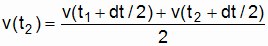. (7_2)

In general we speak of the velocity at some particular moment t, and the same goes for acceleration. But as previously mentioned, we should never entirely forget that any measurement of velocity really entails 2 measurements of position, however close they may be made in time. Similarly, any measurement of acceleration necessarily entails 3 measurements of position.

We could keep going, and define da/dt by making 5 measurements of position, etc. However, for present purposes it will suffice that we know what velocity and acceleration entail.

One of the most obvious examples of acceleration in the natural world occurs when we drop an object from some height. The object accelerates toward the ground. Galileo was first to discover that this acceleration is constant. At the moment of release the object is at rest. A short time later it is falling with a certain speed; it has accelerated downward. A short time later it is falling at an even greater speed; its speed is constantly increasing (i.e., the object is constantly accelerating downward). The wondrous thing about free-falling objects is that, if the difference in height of the beginning and end points are not too great, then the acceleration is constant over the whole length of the fall!

Chapter 8

Force

Points in space, moments in time, velocity and acceleration … all of these are elements of geometry and kinematics. In this chapter we introduce the first truly physical quantity. It is pivotal to that branch of physics known as "mechanics," as first enunciated by Isaac Newton.

Force, like time, is one of those quantities we seem to know about intuitively, but which we might find it hard to define precisely. The late Richard Feynman commented (somewhat waggishly) that force is how hard we push or pull on something. More generally, it is the push or pull that one object in the physical world exerts upon another.

Again like time, we must trust our instincts when it comes to measuring force. For example, 2 bricks feel twice as heavy as one brick; so we assume that gravity pulls the two together with twice the force of one. (Indeed this belief forms the basis of pan balances used to measure the weights of other things.)

Robert Hooke, a contemporary of Newton, discovered that the magnitudes of the forces exerted by the ends of a stretched or compressed string are proportional to the amount of stretch or compression. Thus a spring, say one with one end anchored to something immovable, can be used to exert a force of known size upon something attached to its other end. According to Hooke’s force law,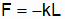, (8_1)

where k is a constant characteristic of the spring. (Stiff springs have large spring constants; limp springs have small ones.) L is the amount of stretch or compression of the unfixed end from the unstressed position. Note the minus sign in Eq. 8_1; a stretched spring tends to contract; a compressed spring tends to expand.

Isaac Newton discovered another force law of great importance. He discovered that the force of gravitational attraction between two objects is proportional to the product of their masses and inversely proportional to the square of their separation. In equation form,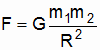. (8_2)

According to Newton the force of gravity between 2 objects is always attractive. "G," the constant of proportionality, is "universal": it works equally well for all known bodies.

But what is this thing called "mass?" Roughly speaking, we can say that mass is the quantity of "matter" in an object. That, of course, begs the question about what "matter" is. It is a profoundly interesting question, and we shall not delve into it here. Suffice it to say that we believe the mass of 2 identical objects is twice that of either one alone. And we know experimentally that material bodies attract each other. If we know what the gravitational proportionality constant is (and we do, to great accuracy), then we can find the masses of two objects by measuring the attractive force between them.

Unfortunately, the force of gravity between everyday objects … objects that we can handle … is very slight. Compounding the problem is the fact that there are countless objects … the Sun and Earth, the Earth and its Moon, etc., that we can’t handle. We must use other methods to determine their masses.

Since we believe that we know the mass of the Earth, one way to determine a manageable object’s mass is to weigh it. We can often do this using a spring, a la Hooke. For something too large to hang from a spring (say the Moon), we must use other methods. Believe it or not, such methods are known. Newton’s laws of mechanics provide the needed tools.

Chapter 9

Newton’s First Law

Newton’s First Law states that "insofar as the force acting on a body is zero, the body remains at rest or moves in a straight line at constant speed." Actually Newton didn’t discover this law; Galileo (his predecessor) did.

If you think about it, you can see right away that there must be something more to Newton’s 1st law than what the words say. For let’s imagine that we are out in deep space, far removed from every other object, and we are observing a small particle which we measure to be at rest (zero velocity). (We assume that our own mass is so slight that the force of gravity we exert on the small particle is practically zero.) Thus far Newton’s 1st law seems to be working fine.

If we accelerate a little bit, so that we change our own velocity (or state of rest) relative to the particle, then relative to us the particle now moves in a straight line with a constant speed (and relative to the particle we move in the opposite direction at the same constant speed). Here again, Newton’s 1st law seems to be working just fine.

But what about the time when we were actually accelerating? During that time and relative to us, the particle was accelerating in the opposite direction! Yet no force was acting on it! Has Newton’s 1st law failed? Clearly if it is to be valid then we must qualify things in some way.

The key to this conundrum is that Newton’s laws work only as the motions of objects are measured from inertial frames of reference. When we are accelerated in the example above, things are not the same as when we are not accelerating. When we are accelerated we feel some accelerating agent (perhaps a rocket thruster) pushing against us in the direction of our acceleration. The frame of reference we remain at rest in while accelerating is a non-inertial frame of reference. And Newton’s laws do not work in non-inertial frames of reference. Once we stop being accelerated, the force of whatever was pushing on us disappears, and we are again back in an inertial frame of reference. And once again Newton’s 1st law applies to the particle.

Newton’s 1st law is thus actually giving us the ground rules for determining which frames of reference are inertial and which ones are not. The rule is that if a particle obeys Newton’s 1st law, then we are observing the particle from an inertial frame of reference. If the particle accelerates when no force acts on it, then we are observing the particle from a non-inertial frame of reference.

But what about when a force does act on the particle, and we are observing it from an inertial frame of reference. When the rocket thruster acted on us, we accelerated. And as you might guess, the particle will similarly accelerate when a force acts on it. This leads us into Newton’s astounding 2nd law … a law that changed the history of mankind in profound ways.

Chapter 10

Newton’s Second Law

Newton noticed that (viewed from an inertial frame of reference) a particle’s acceleration is proportional to the force acting on the particle. If the acting force is doubled, then the acceleration doubles, etc. Furthermore, the proportionality constant is itself proportional to the object’s mass. If the mass is doubled, the proportionality constant is doubled. Newton decided that, since the proportionality constant is itself proportional to the mass, he might just as well say that the mass is the proportionality constant. His 2nd law is accordingly written as: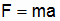. (10_1)

After Newton enunciated this law, all manners of physical phenomena became explainable. Brahe and Kepler (predecessors of Newton) had carefully plotted the motions of the planets around the Sun. Suddenly these motions were understandable as natural consequences of Newton’s 2nd law. The general approach was: (1) apply Newton’s gravitational force law to determine the force the Sun exerts on a planet; (2) apply Newton’s 2nd law to determine the effect of that force on the planet’s motion.

Another whole area of inquiry was to apply Hooke’s spring law to particles, in combination now with Newton’s 2nd law. Suddenly the oscillatory motions of objects dancing up and down on the ends of springs was explainable! The whole area of dynamics (moving under the influence of forces) became open to analysis and experimentation.

As mentioned above, if the force on an object is doubled, then its acceleration doubles. The other side of Newton’s 2nd law is: if the same force is applied to two objects, where one object’s mass is twice that of the other, then the more massive object’s acceleration will be half that of the less massive one’s.

It is important to note that Newton didn’t say that ma is proportional to F. He said that ma equals F. This builds an equality between physical quantities that had hitherto been considered distinct. That is, the "newton" (a popular unit of force) is actually equivalent to a kilogram accelerating at a rate of 1 meter per second squared: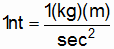. (10_2)

(Here "nt" stands for "newton," "kg" stands for "kilogram", and "m" stands for "meter." "sec" of course stands for "second.") In other words,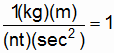, (10_3)

and the trick of multiplying a physical quantity by 1, one or more times, is enriched in a hitherto unexpected way.

The equivalence of force and mass times acceleration, as enunciated by Newton, has a rather interesting consequence in the area of cause and effect. According to Newton, a force exerted on an object results in a perfectly definable acceleration. But the equality works both ways! If a moving object bumps into a resting one, then the moving object will decelerate (or slow down) and exert a force on the second object according to Newton’s 2nd law. But of course if the object decelerates, then it must be that a force is being exerted upon it! Evidently it’s impossible for one thing to exert a force on another, without the other exerting a force back on the first. Newton enunciated this phenomenon in his 3rd law. In modern terms: for every force that one object exerts on another, the other object exerts an equal and oppositely directed force on the first. The total force exerted upon any two interacting objects is zero! We shall investigate some of the ramifications of this remarkable fact in due course. But first we must distinguish the processes (1) where a force acts for some period of time from (2) where a force acts over some distance.

Chapter 11

Work and Impulse

Let us suppose that a particle moves along the x axis of our inertial frame and that a force acts in the same or opposite direction as the particle’s velocity. In an infinitesimal time the force will act over an infinitesimal distance. There is a special name for the product of a force times the distance through which it acts. It is called "work:" work equals force times distance.

The velocity’s magnitude (or the particle’s speed) is just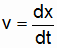, (11_1)

and the infinitesimal distance traveled is thus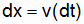. (11_2)

The (infinitesimal amount of) work done is thus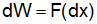. (11_3)

Or, invoking Newton’s 2nd law: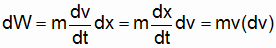. (11_4)

If we add up an infinite number of back-to-back dW’s (or, in the language of calculus, if we integrate) to obtain some total finite amount of work done, then we get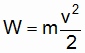. (11_5)

(Here we have assumed for convenience that the initial speed, when the force was first brought to bear, was zero.)

There is a special term and abbreviation for the quantity (mv2)/2. It is called kinetic energy and it is often abbreviated as T. In words, Eq. 11_5 says the work done by a force, acting through a distance, equals the change in kinetic energy (DT) of the particle upon which it acts. This important result is generally referred to as the Work Energy Theorem.

Let us now consider the case where the force acts for some infinitesimal interval of time. The product of force times time is called "impulse" which we can denote as "I":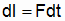. (11_6)

Again invoking Newton’s 2nd law we have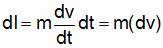. (11_7)

And again adding up an infinite number of back to back infinitesimal impulses, we find that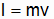. (11_8)

Like mv2/2, mv has a special name and abbreviation. It is called momentum and is abbreviated as P. In words, Eq. 11_8 says the impulse done by a force, acting over some time, equates to the change in the momentum (DP) of the particle being acted upon. This statement is the equivalent of the Work Energy Theorem. It might be called the Impulse Momentum Theorem.

Summarizing, then,

Work = Force X Distance = Change in Kinetic Energy (DT),

Impulse = Force X Time = Change in Momentum (DP).

Chapter 12

Newton’s 3rd Law and the Conservation of Energy and Momentum

According to Newton’s 3rd law, whenever an object exerts a force on another object, then the second object exerts an equal and oppositely directed force on the first. The Sun pulls on the Earth with a gravitational force. But it’s a 2-way street; the Earth exerts an equal and oppositely directed gravitational force on the Sun. (The Sun doesn’t accelerate nearly as much as the Earth does, since the Sun is so very much more massive than the Earth.)

Let us label the masses of the two interacting objects m1 and m2. Again for convenience we’ll suppose that the 2 objects move along the x axis. Let F1,2 be the force that m1 exerts on m2. The infinitesimal change in momentum that m2 experiences in an infinitesimal time dt is just F1,2dt.

Now according to Newton’s 3rd law, m2 also exerts a force on m1, say F2,1. And this force is equal but oppositely directed to F1,2: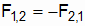. (12_1)

In the same infinitesimal time period, then, m1 has an equal but oppositely directed change in momentum as m2 has. In any finite time interval … in any sum of an infinite number of back-to-back dt’s … the total change in momentum of this system of 2 interacting particles is zero! The total momentum of a system of interacting particles is conserved; it does not change with the passage of time. This is the first of the 2 great conservation laws; it is called the Conservation of Momentum law.

Let us now imagine that the two particles are in contact with one another, with m1 again exerting the force F1,2 on m2 and vice versa. When the mutual distance traveled is dx, then the work done by F1,2 is F1,2dx. And, since F2,1 = -F1,2, we have F2,1dx = -F1,2dx. The total work done by the 2 forces is zero. Or, by the Work Energy Theorem, the total change of kinetic energy, in any system of interacting particles, is zero. This is a statement of the Conservation of Energy Law. It is noteworthy that this law is obeyed even when the particles are not in contact with one another, say when they interact gravitationally.

Virtually any resting object that we can see with the naked eye is composed of millions of atoms. These atoms vibrate about millions of points, doing a complicated dance. Since momentum is a vector, all of their individual momenta at any moment essentially sum to zero … the momentum of the overall object is zero. However, kinetic energy is not a vector; the myriad kinetic energies do not sum to zero. They sum to some value that is proportional to their mean kinetic energy, and this sum is generally referred to as heat energy. Although the object as a whole may be standing still, it contains a certain amount of internal kinetic or "heat" energy.

We can gain an appreciation of the utility of the conservation laws by considering the case where 2 objects, say 2 equal-mass lumps of clay, collide and stick together. Let us suppose that before the collision the lumps are traveling with equal and oppositely directed velocities along the x axis. Their total momentum is zero. What will the speed of the super lump be following the collision? According to the Conservation of Momentum law, the final total momentum must equal the initial total momentum. Thus following the collision the super lump is at rest!

But the initial kinetic energy … the initial motional and internal (heat) kinetic energy … is just twice that of either lump alone. Following the collision the total energy must be the same as before the collision. Yet the super lump is at rest … the final motional kinetic energy is zero! How can this be? The answer lies in the heat energy generated by the collision. Although the super lump may be at rest following the collision, the mean vibrational kinetic energy of its constituent atoms is greater than that of the 2 lumps prior to the collision! Indeed the gain in vibrational kinetic energy equals the loss of motional kinetic energy of the two lumps.

There is a particular parameter used to express the mean vibrational kinetic energy of the atoms in an object; it is called the object’s "Temperature." The greater the mean vibrational kinetic energy, the greater the object’s temperature. Following the collision of our two lumps of clay, the temperature of the final super lump is greater than the (equal) temperatures of the 2 lumps prior to the collision.

As you can imagine, just knowing what the vibrational motions are (and hence what the total vibrational kinetic energies are) at any point in the above-described experiment would be a daunting (and generally impossible) task! All we need do, however, is invoke the Conservation laws to understand (1) why the super lump is finally at rest, and (2) why it is at a higher temperature following the collision. We need not fret about the "devil" in the details. The conservation laws are of enormous utility in analyzing many physical problems. And they are a grand bonus that trace directly back to Newton’s 2nd and 3rd laws!

Chapter 13

Potential Energy

In Newton’s laws "force" refers to the total force acting on an object. Here is an example where the total force equals zero: an athlete stretches a spring by pulling on handles attached to the spring’s ends. Since force is a vector, two equal magnitude but oppositely directed forces sum to zero. And since the total force acting on the spring is zero, its momentum remains zero.

Now work is not a vector. Each of the athlete’s hands does a certain amount of work in stretching the spring. The spring’s velocity is zero once it’s been stretched, so these combined works couldn’t have changed the motional kinetic energy. And we know that the spring doesn’t get hot … its internal kinetic (or heat) energy hasn’t increased (at least not much). Where did the work done by the athlete’s hands go?

The answer is that this work is manifest as potential energy in the spring. By "potential" it is meant that the stretched spring has the potential to do work. It can do this work on whatever it is attached to when it’s allowed to return to its unstretched state.

This simple example not only illustrates potential energy; it illustrates the concept of negative work. When a force acts in the same direction as a change in displacement of the object it acts on, then it does positive work. But when it acts opposite to the change in displacement it does negative work. As the athlete stretches the spring his hands move outward. They do positive work on the spring. But (remember Newton’s 3rd law) the spring pulls inward on his hands and does negative work on the athlete while it’s being stretched. You can see that the total work done on the athlete and the spring is zero! In stretching the spring the athlete expends energy and the spring gains exactly an equal amount of potential energy. When the athlete allows the spring to return to its unstretched state the situation is reversed. Because of the stretching of the spring when it is elongated, the potential energy in this case is called elastic potential energy.

There is also potential energy associated with an object’s position in a gravitational field. It is of course called gravitational potential energy. Let’s say a boy lifts a ball up off the ground to some height h. The ball is initially and finally at rest; there is no net gain in kinetic energy. Yet the boy has done (positive) work. That work equates to a gain of gravitational potential energy of the ball/Earth system. The ball can do work on whatever allows it to return to the ground.

What if the boy drops the ball from the height h? The ball’s kinetic energy does increase as it accelerates downward toward the ground. As you might guess, the gain in kinetic energy exactly equals the loss in gravitational potential energy at each point along the fall. The total energy remains constant … it is conserved.

How about if the ball is on a string, as illustrated in Fig. 13_1.

Figure 13_1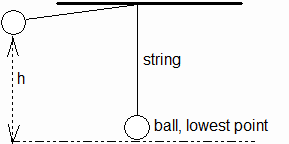Ball Moves in Curve

Initially the energy is all gravitational potential. But when the ball reaches the lowest point some of the potential energy will have been converted to kinetic energy. How much? According to Newton, the gravitational force downward is just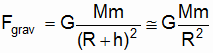. (13_1)

Here M is the Earth’s mass, m is the ball’s mass, and R is the Earth’s radius. Thus at a distance h above the ground the potential energy (denoted as Ugrav) is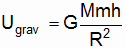. (13_2)

And at its lowest point this will be the ball’s kinetic energy: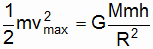. (13_3)

The ball’s speed at this lowest point will therefore be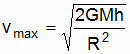. (13_4)

Note that m has dropped out of the result for vmax. Any object would have the same maximum speed at the bottom of the swing … an amazing fact first discovered by Galileo.

Perhaps you can see what a powerful problem-solving tool the Conservation of Energy law can be when both the potential and kinetic forms of energy are taken into account. We can play the same sort of game with the potential energy of a spring, and the kinetic energy of a weight bouncing up and down on the end of the spring. In this case we need only bear in mind that there are two forms of potential energy … gravitational and elastic … in addition to the weight’s kinetic energy.

Chapter 14

P, T and Changing Frames

The formulas for momentum and kinetic energy are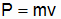, (14_1)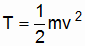. (14_2)

Both P and T contain a "v" term (velocity or speed). But velocity is a relative matter; it depends on which frame of reference one is observing things from. Let us illustrate. In inertial frame K a particle moves in the positive x direction at constant velocity v. It has the momentum and kinetic energy specified by Eqs. 14_1 and 14_2. But what is the situation from the perspective of frame K’, which moves in the positive x direction of K at speed v? In K’ the particle is at rest. It has zero momentum and zero kinetic energy! Clearly P and T are not intrinsic properties of a particle; they depend on one’s selected frame of reference!

Fig. 14_1 depicts a ball oscillating on a spring that is anchored to an immovable wall in inertial frame K. At the moment shown the ball is moving to the left at its maximum speed vmax.

Figure 14_1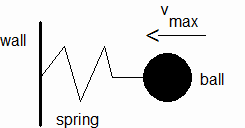Oscillating Ball, Frame K

A short time later the ball will have moved to the left and come to a halt, compressing the spring in the process. The ball will have lost kinetic energy in the amount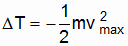. (14_3)

And the spring will have gained potential energy (U) in the amount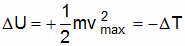. (14_4)

(Notice that DT + DU = 0 … Conservation of Energy.)

But let us now view things from a second frame K’, moving to the left at speed vmax. At the moment depicted in Fig. 14_1 the ball is at rest and the spring moves to the right at speed vmax. Of course the spring is relaxed at the moment and its potential energy is again zero: U’init=0. But now the ball’s initial kinetic energy is also zero: T’init=0.

Again a short time later (and now from the perspective of K’) the spring will be compressed. And the ball will be moving to the right at speed vmax. Once again we will have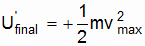. (14_5)

But now we will also have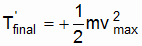. (14_6)

From the perspective of K’,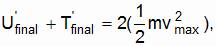(14_7)

Has Energy Conservation been violated? No! We must realize that the "immovable" wall in frame K moves to the right in K’ at speed vmax. And as the spring compresses in K’ it exerts a force on the wall. The wall reciprocates (Newton’s 3rd law) and, in K’, does work. As you might guess (thanks to Energy Conservation),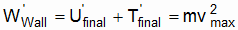, (14_8)

and energy is indeed conserved.

The moral of this little problem is clear. One should always take care to identify all of the forces "at work" when a change of reference frame is contemplated. A force that does no work in frame K will often do work in K’. Like the changes in potential and kinetic energies that occur when we change frames, the work(s) done by the force(s), when viewed in K, may be different from the work(s) done by the same force(s) when things are viewed from frame K’.

Chapter 15

Transformations

What we need at this juncture is a method to transform quantities from one inertial frame to another. Say frame K’ moves in the positive x direction of K at constant speed u. Let us begin with force. In Newton’s mechanics force, like elapsed time, is a matter of agreement among all inertial observers: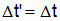(15_1)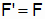. (15_2)

Similarly, everyone agrees on a particle’s mass: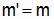. (15_3)

To the extent Newton’s laws are "covariant" (i.e., the laws are equally applicable in all inertial frames), Eqs. 15_2 and 15_3 suggest that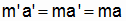, (15_4)

and thus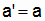. (15_5)

Like force, acceleration is invariant under a transformation.

As one might imagine, velocity is quite another matter. In the case of motions confined to the x axis, we find that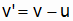. (15_6)

(Special case: v=u so that v’=0). Expressing Eq. 15_6 in terms of dx and dt: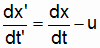. (15_7)

And multiplying through by dt produces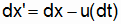. (15_8)

Or, adding up an infinite number of the differentials (i.e. of the infinitesimal quantities):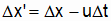. (15_9)

The work done by F, acting through dx, is. (15_10)

Let us in this case divide through by dt: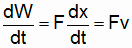. (15_11)

dW/dt is the rate at which F does work. It is called "power." From the Work Energy Theorem we find that (1) the rate at which a particle’s kinetic energy changes (2) equals the power expended by the acting force: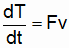. (15_12)

Note that if the force is acting on an anchored spring, then it is the spring’s potential energy that varies in time: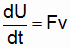. (15_13)

As we saw in the last chapter, dT/dt is a relative matter, quite as v is. An "immovable" anchor in one frame will move at a constant velocity in another. Any zero-power force that the anchor exerts in its rest frame may transform to nonzero power in the frame where the anchor moves.

Chapter 16

Center of Mass

The solid objects (and, yes, the containers of gas) that Newton experimentally found to be so well behaved consist in reality of countless atoms doing complicated dances. The specifics of these dances are impossible to ascertain at any given moment. Indeed even the individual atoms have parts (and so on!) How is it that Newton and his heirs have found such complex conglomerates to behave en masse in such predictable ways?

The answer lies in a stunning and simple fact about objects whose constituent parts interact with one another according to Newton’s 3rd law. There is a geometric point in any such assembly which, to external observers, moves as if the total mass of the parts existed at that point. In effect, to an external observer the entire object behaves like a single particle!

By way of example, let us consider the Earth and its Moon. Although we might be accustomed to think of the Earth as being at rest in some inertial frame, with the Moon orbiting it in an ellipse, the reality is that the Earth and the Moon mutually orbit about a single point known as the center of mass. Owing to the Earth’s much greater mass, this center of mass lies within the globe’s spherical volume, and the Earth’s "orbit" around it amounts to a slight wobble. But that wobble is definitely manifest. Together with the Moon’s gravitational tug and the fluidity of water, it accounts for the oceanic tides.

Viewed from the Sun’s rest frame, it is not the Earth that orbits the Sun in an elliptic path; it is the center of mass of the Earth/Moon system. And in applications of Newton’s 2nd law it is the summed masses of the Earth and the Moon, which should be used. We "get away" with using only the Earth’s mass because of that astounding fact discovered by Galileo: that objects of diverse masses all "fall" (i.e. accelerate) at the same rate in a gravitational field.

From the Earth/Moon system we can move on to grander stages, or we can turn inward to more humble ones. In either direction every system of interacting parts behaves, en masse, as though it were a single particle at the system’s center of mass. Viewed from the galactic rest frame, for example, the center of mass of our entire Solar System moves along a smooth path in accordance with Newton’s 2nd law. But here again the Sun wobbles slightly; the planets wobble under the tugs of their Moon(s), etc. Yet the entire Solar System’s center of mass moves with this elegant simplicity.

Perhaps one of the most dramatic examples is an artillery shell that explodes in flight. Prior to the explosion the trajectory is perfectly predictable using Newton’s laws. No big surprise here. But after the explosion, fragments fly away in all directions. Some of them even go "backward" faster than the shell’s pre-explosion speed! Prior to the explosion no one can predict precisely what the velocities of the fragments will be. Yet we can predict precisely what the exploded system’s center of mass will do, regardless of the outcome’s details. The center of mass will continue on along the same path that the shell would have followed, had the explosion never occurred! It is a beautiful and enormously useful result, long-used by savvy artillery officers (and, more recently, by their computers).

Chapter 17

Every vector such as velocity has a magnitude and a direction. And as discussed, acceleration is the time rate of change of velocity. In particular we have considered the case where a velocity’s magnitude changes with time. In this chapter we consider cases where the direction changes with time.

The simplest example is perhaps when a particle travels in a circle at constant speed. Its velocity vector points tangent to its point on the circular path at all times. Whereas the vector’s length doesn’t change with time, however, its direction clearly does. Fig. 17_1 illustrates the velocity at two closely spaced instants in time.

Figure 17_1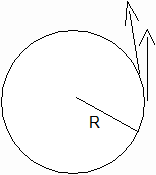Velocities at 2 Closely Spaced Instants

Let us see what Dv must be, where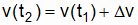. (17_1)

We can depict Dv graphically by redrawing v(t1) so that its tail coincides with the tail of v(t2). Fig. 17_2 illustrates.

Figure 17_2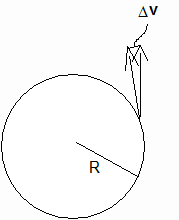Dv

As you can imagine, when t1 and t2 are practically equal, (1) the magnitude of Dv gets very small, and (2) Dv points toward the center of the circle.

The fact that the magnitude of Dv becomes small as t2 approaches t1 is no cause for concern, since t2-t1 is also shrinking, and we are interested in their ratio: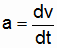. (17_2)

It isn’t difficult to figure out what the magnitude of this ratio is: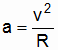. (17_3)

Here then is the result we are seeking. When a particle goes in a circle at constant speed, its acceleration vector points toward the circle’s center and has a magnitude of v2/R.

This type of acceleration is called radial acceleration because it points along the radius. It is always at right angles to the velocity vector v and hence denotes only changes in the velocity vector’s direction. In general a particle may simultaneously have a mix of linear acceleration (changing speed) and radial acceleration (changing direction).

According to Newton’s 2nd law there must be a force whenever a is nonzero. And indeed a force must be applied to keep a particle going in a circle at constant speed. The force must always point toward the circle’s center. And quite elegantly Newton’s 2nd law works equally well in the case of radial acceleration: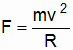. (17_4)

Sometimes this force is called a centripetal force, "centripetal" being Latin for "seeking the center."

If the particle’s path is not circular but is curved, then there will still be "radial" acceleration. In this case the idea is to take a tiny section of arc about the particle and ask, "If this were an arc of a perfect circle, then what would the circle’s radius be?" The answer to that question is called "the radius of curvature," and is the value used in v2/R.

In conclusion, acceleration comes in 2 varieties: (1) linear acceleration, where the velocity magnitude varies in time, and (2) radial acceleration, where the velocity direction varies in time. In general a mix of both kinds of acceleration may be present. An example would be a planet in an elliptic orbit around the Sun. It is obviously traveling along a curved path. But in general its speed is also varying in time.

Chapter 18

Rotational Kinetic Energy

Although the center of mass of extended objects often behaves like a particle, extended objects are in fact not particles. For one thing extended objects can rotate about an axis. (Very often it is convenient to assume that this axis passes through an object’s center of mass.)

Let us consider the Earth from the perspective of its center of mass rest frame. Since momentum is a vector, all of the planet’s atomic momenta sum to zero, and the planet’s net linear (or translational) momentum is zero.

Energy, however, is not a vector; it is expressible as a single quantity (known as a "scalar" in mathematical terminology). As it moves around the planet’s rotation axis, each atom adds a minuscule amount of kinetic energy to the Earth’s net rotational kinetic energy. In the case of the Earth, the amount of this rotational kinetic energy is enormous, owing to the planet’s great mass. (Even though it rotates on its axis only once very 24 hours, the speed of matter at the Equator is 1000 miles per hour!) The Earth’s rotational kinetic energy is a huge repository of energy waiting to be tapped into, if only someone could figure out how to do that.

But how shall we calculate a spinning object’s rotational kinetic energy? In the case of translational kinetic energy we need only know mvcm, an object’s net mass and the speed of its center of mass. The translational kinetic energy is then just mvcm2/2, where m is the object’s entire mass.

There is a similar solution in the rotational case. Every object, spinning around some axis of rotation, has a certain moment of inertia, often denoted as "I" (not to be confused with impulse). And Trotation, the rotational kinetic energy, is just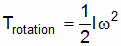. (16_1)

In this equation w is the magnitude of the angular velocity. Note the similarity to the equation for translational kinetic energy: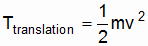. (16_2)

As one might hope, the total kinetic energy of an object that both translates and spins about an axis through its center of mass is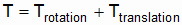. (16_3)

It should be noted that rotational kinetic energy is not classically relative; it does not vary from inertial frame to inertial frame. If an object is spinning in one inertial frame (say in the rest frame of its center of mass), then it is spinning in all inertial frames. Of course it would not spin in its rest frame, but in the case of spinning objects the rest frame is never an inertial frame. In brief, if the object’s rotational kinetic energy is Iw2/2 in its center of mass rest frame, then that is the rotational kinetic energy in every inertial frame of reference.

Chapter 19

Angular Momentum

Although a spinning object has no translational momentum when its center of mass is at rest, it can (like a translating object) exert a force. For example, Fig. 19_1 depicts a spinning flywheel with a rope wrapped around its shaft. The rope is lifting a weight by counteracting the force of gravity.

Figure 19_1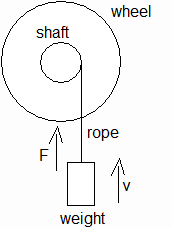Spinning Wheel Exerting a Force

Evidently the flywheel in Fig. 19_1 has some sort of momentum, even though its center of mass does not translate. The momentum in question is associated with the wheel’s rotation, and is called angular momentum. It is often denoted as L, and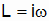, (19_1)

where I is again the moment of inertia and w is the angular velocity. (In Fig. 19_1, w and L point out of the plane of the figure.) Note that Eq. 19_1 is the rotational analog of. (19_2)

In order to provide an angular acceleration to the wheel, one must exert a torque. Torque is the twisting analog of force. Indeed the rotational equivalent of Newton’s 2nd law is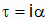, (19_3)

where t is the torque and a is the angular acceleration (or time rate of change of w).

Angular momentum around some reference point can be generalized to particles, planets, etc. If the parts of such a system interact in accordance with Newton’s 3rd law, then the total angular momentum is conserved. Barring outside interaction, the angular momentum will not vary in time.

As in the case of kinetic energy, a key difference between angular momentum and translational momentum is that angular momentum does not vary from frame to frame. If an object has a nonzero L in one inertial frame, then it has the same angular momentum in all inertial frames.

Despite this similarity to kinetic energy, it is not true that an object’s total momentum equals the sum of its translational and its angular momenta. So far as momentum is concerned, the translation of an object’s center of mass is one thing; the object’s rotation about an axis is quite another.

The conservation of angular momentum constitutes a third conservation law. Like the conservation of translational momentum, it is of great use in solving many classical problems. Furthermore, it plays a key role in quantum physics … the physics of atoms and other tiny systems.

Chapter 20

Rockets

The principle of rockets is very simple: throw something out of the back of your ship, and you and what’s left of the ship will accelerate in the forward direction. What is thrown away is usually hot gas resulting from the explosive combustion of liquid or solid fuel.

The easiest way to understand rockets (and jets) is to invoke momentum conservation in the ship’s momentary inertial rest frame: backward momentum imparted to exhaust gas = forward momentum imparted to ship.

Translational momentum and kinetic energy are related by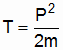, (20_1)

so that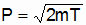. (20_2)

Evidently the greatest momentum that can be attained, by ejecting a mass out of the exhaust, is to give the exhaust gas molecules the greatest kinetic energy. One should accordingly use the most explosive fuel manageable.

As fuel is ejected, the mass of the ship, plus any remaining, unburned fuel, steadily decreases. This means that a rocket not only accelerates, but its acceleration increases with time.

The tank that holds a rocket’s fuel is useless once all the fuel in it has been burned. Thus (assuming there is still fuel in other tanks to be burned) the acceleration can be improved by disengaging empty tanks. That is why big rockets are often multi-stage.

An interesting consequence of an inverse square gravitational field (including Earth’s) is that the greater a rocket’s speed, once it has used up all of its fuel, the higher it will coast before gravity pulls it to a complete halt. Indeed for any given field there is one speed (known as "escape velocity") at which the coasting rocket will not come to a complete halt until it has coasted all the way out to infinity! Any rocket that reaches escape velocity (or beyond) before shutting down its thruster(s) will not fall back to Earth!

Of course the escape velocity is predicated on the idea that the Earth is the only gravitating body in the universe. In reality as one ventures out into the Solar System the effects of the other planets’ gravitational fields (some of which dwarf the Earth’s field in comparison) will be felt more and more.

In navigating through the solar system, it is possible to increase a rocket’s speed relative to the Sun by skimming past a planet that itself moves in the Sun’s rest frame. This "sling shot" effect lessens the amount of fuel that must initially be burned in order to coast to some other planet of interest. And of course the effect can be reversed to slow down "incoming" rockets upon their return to Earth bases.

Like moving gas molecules, electromagnetic radiation has momentum. And an interesting variation on thruster technology is the emission of radiant energy out of a rocket’s tail. This results in a slight reaction force acting on the ship in the forward direction. The acceleration is not nearly as great as that realizable by ejecting hot gas. But the efficiency … the amount of final momentum/velocity per kilogram of "fuel" … is considerably greater. In cases where ships must take off and land on planet surfaces and make long voyages through more or less gravity-free space, hybrids of gas-fired and radiant energy thrusters may be the way to go.